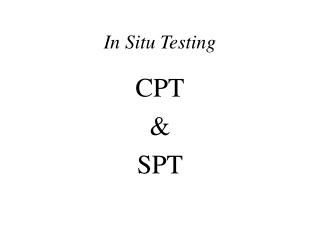DownloadDownload PresentationIn Situ Testing

In Situ Testing

Download PresentationIn Situ Testing

- - - - - - - - - - - - - - - - - - - - - - - - - - - E N D - - - - - - - - - - - - - - - - - - - - - - - - - - -
Presentation Transcript

1. In Situ Testing CPT & SPT

2. CPT Testing

3. CPT Testing Three Measurements qC = Cone-tip resistance uP = Penetration pore pressure (piezocone) fS = sleeve friction Ratios FR = friction ratio = fS / qC Bq = pore pressure ratio = (uP-uh) / (qT –  'vo)

4. Sand Clay Crust SCPTu Sounding, Memphis, Tennessee Real-Time readings in computer screen Penetration at 2cm/s

5. qT Corrected cone-tip resistance qT =qc + (1-an)uP only for type 2 (type B) cones an = 0.8 = fn(cone) net area ratio

6. CPT-parameters ´ (Sands) See page 129 fig. 4.16 ´ = 17.6 + 11 log ( qC / (´vo.pa)0.5 ) Kulhawy & Mayne(1990) ´ = arctan[(0.1 +0.38 log (qC / ´vo))] qC1= qC/(´vo.pa)0.5

7. CPT-parameters Dr = relative density (sands) Dr = 100 (qC1 / 305·OCR0.2 )0.5  if unknown, use OCR = 1 e = void ratio e = 1.152 – 0.233·log(qC1) + 0.043 log(OCR)

8. CPT-parameters OCR (iterative) Ko = 0.192 ( qC / pa)0.22 (´vo / pa)-0.31 OCR 0.27 (1) Ko = ( 1 - sin´)OCR sin ´(2) (1) Mayne,CPT’95 (2) Mayne & Kulhawy,1982 a)Find ´ b)Vary OCR will both Ko values are similar

9. SPT Testing

10. SPT Testing Procedure 1) Drill boring (hole) 2) Insert SPT sampler (hollow) 3) Drive sampler into the ground 18” & count N of blows to drive each 6” interval if N for 6” > 50 if N for all 18” >100 Refusal

11. SPT Testing 4) NSPT = N for last two 6 “ intervals 5) Retrieve sampler & save soil 6) Drill to next depth & go to step 2 NSPT = fn (Method of drilling, how clean boring is, hammer location, hammer type, “hangover level” of technician……..) see p.117-118

12. SPT Testing Efficiency corrections: From “ NSPT” to “N60” N60 = (E mCBCSCRNSPT) / .60 Em = hammer efficiency = fn (hammer type )  Table 4.3 P.119 CB = borehole dia. correction - table 4.4 Cs = sampler correction - table 4.4 CR = rod length correction - table 4.4

13. SPT Testing More corrections: NSPT  as   , so pa = 100 kpa (N1 )60  2N 60 N’s have been correlated with everything !! (N1)60 = N60 ( pa/z)0.5

14. cu = undrained strength gT = unit weight IR = rigidity index ' = friction angle OCR = overconsolidation K0 = lateral stress state eo = void ratio Vs = shear wave E' = Young's modulus Cc = compression index qb = pile end bearing fs = pile skin friction k = permeability qa = bearing stress DR = relative density gT = unit weight LI = liquefaction index ' = friction angle c' = cohesion intercept eo = void ratio qa = bearing capacity p' = preconsolidation Vs = shear wave E' = Young's modulus  = dilatancy angle qb = pile end bearing fs = pile skin friction SAND CLAY Is One Number Enough??? N